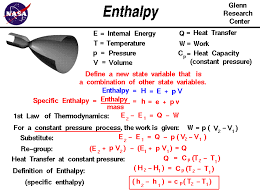Energetics of Chemical reactionThermodynamic terms

In order to study the law of thermodynamics it is essential to understand certain terms used in thermodynamics. These terms have definite meaning. So, the most commonly used terms are

1.    System

A specific portion of matter under study which is isolated from the rest of the universe with a bounding surface is called a system.

2.    Surrounding

The rest of the universe which might be in a position to exchange energy and matter with the system is called the surrounding.

3.    Boundary

Real or imaginary line which separates the system from surrounding is known as boundary.

Homogeneous system and heterogeneous system

A system which is uniform throughout i.e., it has the same chemical composition throughout the system is called homogeneous system. eg, mixture of gases.

A system which is not uniform throughout is called heterogeneous system. It consists of two or more phases which are separated form each other by definite boundary. a system consisting of liquid and its vapors.

Types of systems

Depending upon the boundary, thermodynamic systems are classified in following types:

1.    Open system

A system which can exchange matter as well as energy with the surrounding ids called open system.

Mathematically, for open system

dm ≠ dE ≠ 0 e.g.

Hot Water in a beaker

2.    Closed system

A system that can exchange energy with the surrounding but not the matter is called a closed system.

Mathematically, for closed system

dM = 0 and dE ≠ 0

e.g. Hot water kept in a closed metallic vessel.

3.    Isolated system

A system which can neither exchange matter nor energy with the surrounding is called isolated system.

Mathematically, for isolated system dM = 0 and dE = 0

E.g. Hot water in a thermos

Properties of system

There are two types of observable properties of system, which are:

1.    Extensive properties

The properties which depend upon the amount of the substance present in the system are called extensive properties. For example, mass, volume, energy, work, internal enthalpy, entropy etc.

2.    Intensive properties

The properties which depend upon the nature of substance rather than the amount of substance are called intensive properties. For example– temperature, density, concentration viscosity, refractive index, surface tension, specific heat, pressure etc

Thermodynamic process

The process by which a thermodynamic system proceeds from one state to another is called thermodynamic process. Various types of thermodynamic process are as follows:

1.       Isothermal process

A process in which although heat enters or leaves the system but the temperature of the system remains constant throughout the process is called isothermal process.

For isothermal process , dT = 0

A process during which no heat enters or leaves the system during any step of the process is known as adiabatic process:

For adiabatic process, dq = 0

3.       Isobaric process

A process during which pressure of the system remains constant throughout the process is known as isobaric process.

For isobaric process, dp = 0.

4.       Isochoric process

A process during which volume of the system remains constant throughout the process is known as isochoric process.

For an isochoric process, dv = 0

5.       Cyclic process

A process during which system comes to its initial state through a number of different processes is called a cyclic process.

For a cyclic process, dE = 0, dH = 0.

Internal energy:

A system contains different form of energy such as, kinetic energy, potential energy, rotational energy, vibrational energy, bond energy etc. The total sum of different form of energy associated with a particle in a system is known as internal energy. It is denoted by a symbol E or U.

First law of thermodynamics

The first law of thermodynamics is simply the law of conservation of energy which states that “energy can neither be created nor destroyed although it can be converted from one form to another.”Sign conventions of Heat and works: -

i.                 q is positive if heat is absorbed by the system

ii.               q is negative if heat is lost by the system

iii.              W is positive if work is done by the system

iv.              W is negative if work is done on the system.

State function:

State function is a physical quantity whose value depends only upon the initial and final state of a system but not upon the path by which the state of a system is changed. In other word state functions are path independent but state dependent thermodynamic properties. For example, internal energy, enthalpy, free energy etc.

Reversible and irreversible process:

The process which is carried out very slowly so that a changes occurring in the forward direction can be exactly reversed and the system remains in a state of equilibrium with the surrounding is called reversible process. It may be defined as a process which is conducted in  such a way that at every state driving force is slightly greater than the opposing force and which can be reversed by increasing the opposing force by an infinitesimal small amount.

A process which is completed in a single step and can not be reversed is called irreversible process. In the other words irreversible process is the process in which the successive steps of the direct process can't be reversed by increasing opposing force

﻿